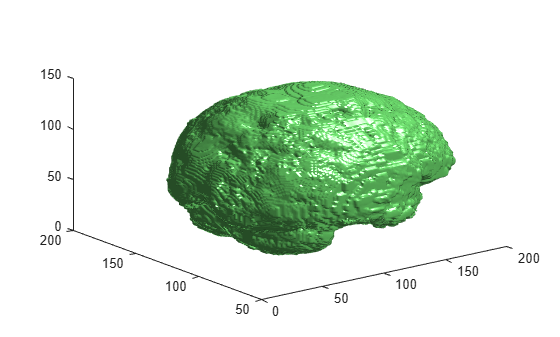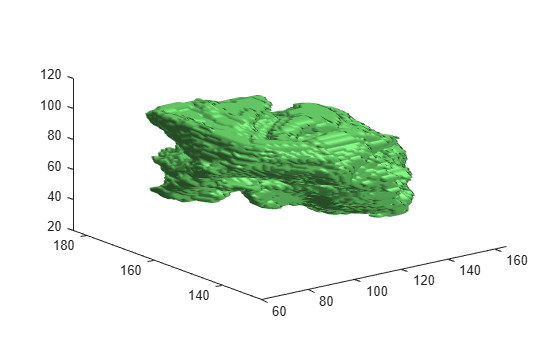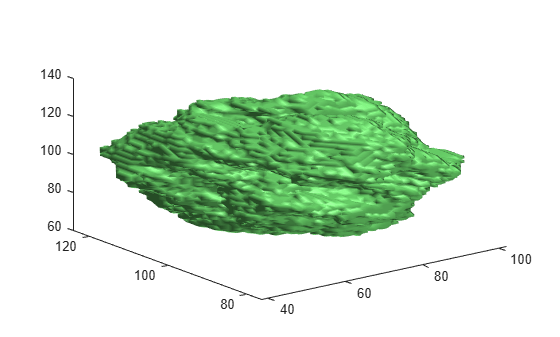# extractIsosurface

Extract isosurface from volume using marching cubes algorithm

## Syntax

``[faces,verts] = extractIsosurface(V,isovalue)``

## Description

An isosurface is a 3-D surface representation of points with equal values in a 3-D intensity volume. The `extractIsosurface` function returns the face and vertex data of the isosurface extracted by connecting points of a constant value within a volume of space. The `extractIsosurface` function uses the marching cubes algorithm to extract isosurface data as arrays faster than the corresponding syntax of the `isosurface` function, without compromising resolution. For additional options, you must use the `isosurface` function.

example

````[faces,verts] = extractIsosurface(V,isovalue)` extracts an isosurface from the intensity volume `V` by determining where the values of `V` are equal to the specified isovalue `isovalue`. The function returns the face and vertex data of the isosurface in `faces` and `verts`, respectively.```

## Examples

collapse all

Load the intensity volume data into the workspace.

```load(fullfile(toolboxdir("images"),"imdata","BrainMRILabeled","images","vol_001.mat")); V = vol;```

Specify the isovalue for isosurface extraction.

`isovalue = 100;`

Extract the isosurface of the input volume at the specified isovalue.

`[faces,verts] = extractIsosurface(V,isovalue);`

Plot the extracted isosurface.

```figure p = patch(Faces=faces,Vertices=verts); isonormals(V,p) view(3) set(p,FaceColor=[0.5 1 0.5]) set(p,EdgeColor="none") camlight lighting gouraud```Load the intensity volume data into the workspace.

```load(fullfile(toolboxdir("images"),"imdata","BrainMRILabeled","labels","label_001.mat")); V = label;```

Specify the isovalue for isosurface extraction.

`isovalue = 0.05;`

Extract the isosurface of the input volume at the specified isovalue.

`[faces,verts] = extractIsosurface(V,isovalue);`

Display and inspect the extracted isosurface.

```figure p = patch(Faces=faces,Vertices=verts); isonormals(V,p) view(3) set(p,FaceColor=[0.5 1 0.5]) set(p,EdgeColor="none") camlight lighting gouraud```Create a point cloud from the vertices of the extracted isosurface. Display the point cloud.

```ptCloud = pointCloud(verts); figure pcshow(ptCloud)```Load the intensity volume data into the workspace.

```load(fullfile(toolboxdir("images"),"imdata","BrainMRILabeled","labels","label_002.mat")); V = label;```

Specify the isovalue for isosurface extraction.

`isovalue = 0.05;`

Extract the isosurface of the input volume at the specified isovalue.

`[faces,verts] = extractIsosurface(V,isovalue);`

Display and inspect the extracted isosurface.

```figure p = patch(Faces=faces,Vertices=verts); isonormals(V,p) view(3) set(p,FaceColor=[0.5 1 0.5]) set(p,EdgeColor="none") camlight lighting gouraud```Triangulate the extracted isosurface. Display the triangulation as a mesh.

```T = triangulation(double(faces),double(verts)); figure trimesh(T)```Create an STL file for 3-D printing, using the triangulation of the extracted isosurface.

`stlwrite(T,"brain.stl")`

## Input Arguments

collapse all

Intensity volume data, specified as a 3-D numeric or logical array.

Data Types: `single` | `double` | `int8` | `int16` | `int32` | `uint8` | `uint16` | `uint32` | `logical`

Isovalue at which to compute the isosurface, specified as a numeric scalar.

Data Types: `single` | `double`

## Output Arguments

collapse all

Face data of the computed isosurface, returned as an M-by-3 matrix. M is the number of faces in the isosurface. Three vertices form a triangular face. The 3-D index coordinates of all the vertices of the surface are returned as the rows of `verts`. Each row of the `face` matrix contains the row indices of the three vertices from `verts` that form the triangular face.

Data Types: `single`

Vertex data of the computed isosurface, returned as an N-by-3 matrix. N is the number of vertices in the isosurface. Each row of the matrix contains the 3-D index coordinates of one vertex of the isosurface.

Data Types: `single`

## Algorithms

The `extractIsosurface` function uses the marching cubes algorithm to extract the isosurface of a volume `V` at the specified isovalue `isovalue`. The marching cubes algorithm uses lookup tables to obtain information about the faces and vertices of the isosurface. The lookup tables enable the `extractIsosurface` function to extract face and vertex data as arrays faster than the `isosurface` function without compromising resolution for large intensity volumes, such as those typically used in medical imaging. Though the purposes of `extractIsosurface` and `isosurface` are similar, there are certain differences in their implementation and output.

• The behavior of `extractIsosurface` and `isosurface` differs for the edge case when an intensity value is equal to the specified isovalue. The `isosurface` function generates a surface between regions with intensities less than or equal to the isovalue and regions with intensities greater than the isovalue. The `extractIsosurface` function generates a surface between regions with intensities less than the isovalue and regions with intensities greater than or equal to the isovalue.

• Except for the edge case, both `extractIsosurface` and `isosurface` generate the same number of vertices with the index coordinates of the vertices matching within a small tolerance. However, the order of the vertices in the output `verts` can be different.

• Except for the edge case, both `extractIsosurface` and `isosurface` generate the same number of faces. However, the actual faces in the output `faces` are different because both algorithms create the same surface using different triangulations of the vertices.

 Lorensen, William E., and Harvey E. Cline. “Marching Cubes: A High Resolution 3D Surface Construction Algorithm.” ACM SIGGRAPH Computer Graphics 21, no. 4 (August 1987): 163–69. https://doi.org/10.1145/37402.37422.